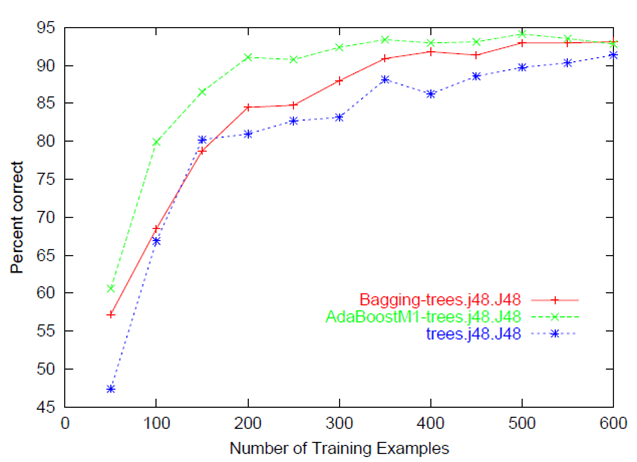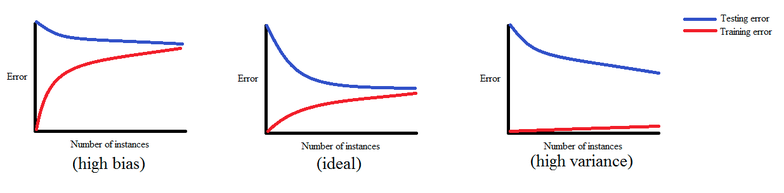# What is a Learning Curve in machine learning?

0 votes
1 view

I want to know what a learning curve in machine learning is. What is the standard way of plotting it? I mean what should be the x and y axis of my plot?

## 1 Answer

0 votes
by (33.2k points)

Learning Curve:

It is used for graphical visualization of the model evaluation process. It usually refers to a plot of the prediction accuracy/error vs. the training set size (i.e: how better does the model get at predicting the target as you the increasing number of instances used to train it)When both the training and test/validation performance are plotted together then we can diagnose the bias-variance tradeoff (i.e determine if we benefit from adding more training data, and assess the model complexity by controlling regularization or number of features).Hope this answer helps.

If you wish to learn Machine Learning visit this Machine Learning Course.

0 votes
1 answer
0 votes
1 answer
0 votes
1 answer
+4 votes
1 answer
0 votes
1 answer